# The QUANTLIFE Procedure (Experimental)

### Nelson-Aalen-Type Estimator for Censored Quantile Regression

Peng and Huang (2008) propose a method for censored quantile regression that is based on the Nelson-Aalen estimator of the cumulative hazard function. Let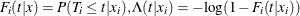, and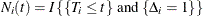. Then the following equation is a martingale process that is associated with the counting process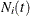(Fleming and Harrington, 1991):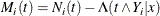Based on the martingale process, Peng and Huang (2008) derive the following estimating equation: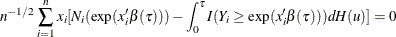where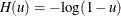and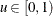. By approximating the integral in the estimating equation on a grid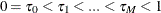, the regression quantiles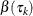,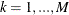, can be estimated sequentially by solving the following linear programming problem: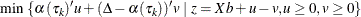where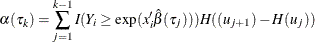See Koenker (2008) for details. You can request this method by specifying the METHOD=NA option. The grid pointsare equally spaced with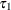specified by the INITTAU=option and the grid step between two adjacent grid points specified by the GRIDSIZE=option.